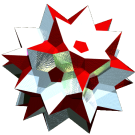Acronym sladid (old: sit) Name small lanceal disdodecahedron,strombic icositetrahedron,deltoidal icositetrahedron,tetragonal icosikaitetrahedron© Inradius sqrt[(78+47 sqrt(2))/68] = 1.457577 Vertex figure [kLL4], [kLS4], [KSS3] Dihedral angles at long edge:   arccos[-(7+4 sqrt(2))/17] = 138.117959° at short edge:   arccos[-(7+4 sqrt(2))/17] = 138.117959° Dual sirco ExternallinksThe kites {(kLS,kLL,kLS,KSS)} have vertex angles k = kLS = kLL = arccos[(2-sqrt(2))/4] = 81.578942° resp. K = KSS = arccos[-(2+sqrt(2))/8] = 115.263174°.

Edge sizes used here are kLSKSS = x = 1 (short) resp. kLLkLS = d = 2-1/sqrt(2) = 1.292893 (long). – The pseudo edge of size e is the symmetric diagonal of the kites, while the pseudo edge of size b is the asymmetric diagonal of the kites.

Incidence matrix according to Dynkin symbol

m3o4m =
aoo3obo4ooc&#z(d,x,e)   → height = 0
a = kLLkLS x(8,2) = sqrt[5+1/sqrt(2)] = 2.388955
b = kLSkLS = a/q = sqrt[10+sqrt(2)]/2 = 1.689246
c = kLSKSS x(8,2) = sqrt[2+sqrt(2)] = 1.847759
d = kLLkLS = lacing(1,2) = 2-1/sqrt(2) = 1.292893
e = kLLKSS = lacing(1,3) = sqrt[3-1/sqrt(2)] = 1.514230

o..3o..4o..         | 6  * * |  4  0 |  4  [kLL4]
.o.3.o.4.o.         | * 12 * |  2  2 |  4  [kLS4]
..o3..o4..o         | *  * 8 |  0  3 |  3  [KSS3]
--------------------+--------+-------+---
oo.3oo.4oo.&#d      | 1  1 0 | 24  * |  2  d
.oo3.oo4.oo&#x      | 0  1 1 |  * 24 |  2  x
--------------------+--------+-------+---
... obo ...&#(d,x)t | 1  2 1 |  2  2 | 24  {(kLS,kLL,kLS,KSS)}